SPSS TUTORIALS BASICS ANOVA REGRESSION FACTOR CORRELATION

# Creating Boxplots in SPSS – Quick Guide

There's 3 ways to create boxplots in SPSS:

• GraphsLegacy DialogsBoxplot
• AnalyzeDescriptive StatisticsExplore
• GraphsChart Builder

The first approach is the simplest but it also has fewer options than the others. This tutorial walks you through all 3 approaches while creating different types of boxplots.

## Example Data

All examples in this tutorial use driving-test.sav, partly shown below.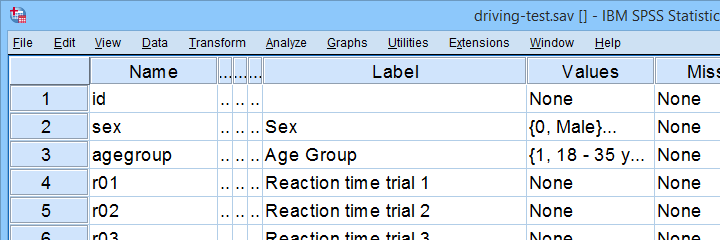Our data file contains a sample of N = 238 people who were examined in a driving simulator. Participants were presented with 5 dangerous situations to which they had to respond as fast as possible. The data hold their reaction times and some other variables.

## Boxplot for 1 Variable - 1 Group of Cases

We'll first run a boxplot for the reaction times on trial 1 for all cases. One option is GraphsLegacy DialogsBoxplot which opens the dialogs shown below.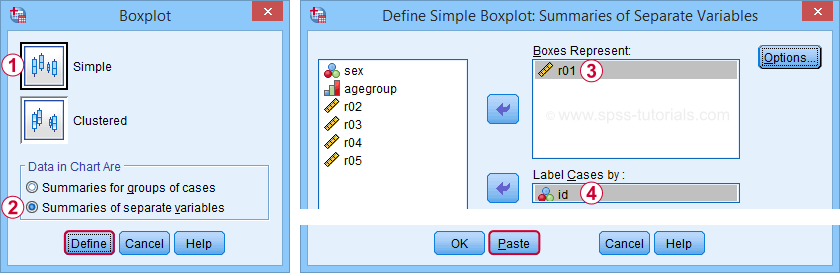Completing these steps results in the syntax below.

*Boxplot for r01 (all cases).

EXAMINE VARIABLES=r01
/COMPARE VARIABLE
/PLOT=BOXPLOT
/STATISTICS=NONE
/NOTOTAL
/ID=id
/MISSING=LISTWISE.

## Result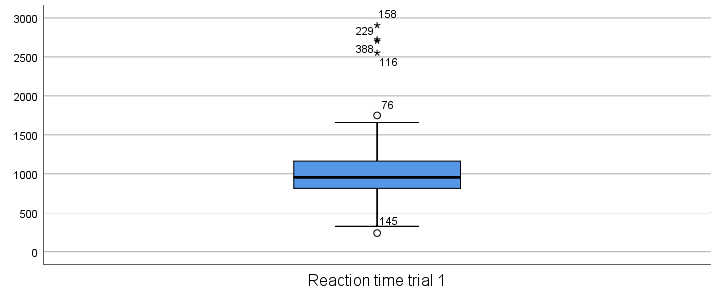Our boxplot shows some potential outliers as well as extreme values. Interpreting these -and all other boxplot elements- is discussed in Boxplots - Beginners Tutorial. Also note that our boxplot doesn't have a title yet. Options for adding it are discussed in Tip 3 - Adding Titles to Boxplots.

## Boxplot for Multiple Variables - 1 Group of Cases

We'll now create a single boxplot for our 5 reaction time variables for all participants. We navigate to AnalyzeDescriptive StatisticsExplore and fill out the dialogs as shown below.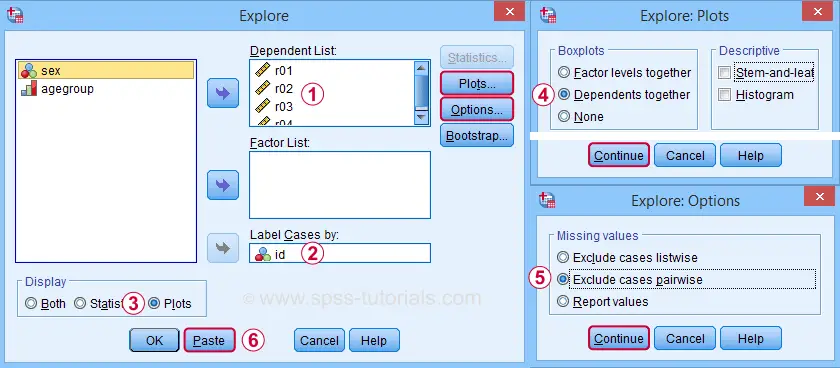“Dependents together” means that all dependent variables are shown together in each boxplot. If you enter a factor -say, sex- you'll get a separate boxplot for each factor level -female and male respondents. “Factor levels together” creates a separate boxplot for each dependent variable, showing all factor levels together in each boxplot.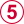“Exclude cases pairwise” means that the results for each variable are based on all cases that don't have a missing value for that variable. “Exclude cases listwise” uses only cases without any missing values on all variables.

A minor note here is that many SPSS users select “Normality plots and tests” in this dialog for running a

Anyway. Completing these steps results in the syntax below. Let's run it.

*Boxplot for comparing 5 variables on 1 group of cases.

EXAMINE VARIABLES=r01 r02 r03 r04 r05
/COMPARE VARIABLE
/PLOT=BOXPLOT
/STATISTICS=NONE
/NOTOTAL
/ID=id
/MISSING=PAIRWISE /* IMPORTANT! */.

## Result

Now, before inspecting our boxplot, take a close look at the Case Processing Summary table first.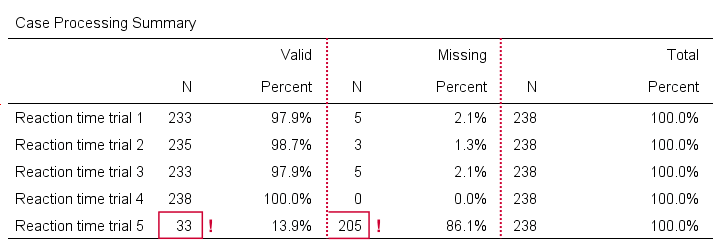The first columns tells how many cases were used for each variable. Note that trial 5 has N = 205 or 86.1% missing values. Remember that “Exclude cases listwise” was the default in the Explore dialog. If we hadn't changed that, then none of our variables would have used more than N = 33 cases. The actual boxplot, however, wouldn't show anything wrong. This really is a major pitfall. Please avoid it.

Anyway, the figure below shows our actual boxplot.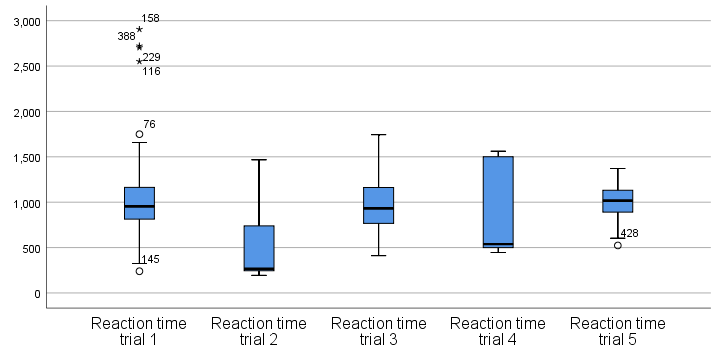Note that we already saw the first boxplot bar in our previous example. Second, trials 2 and 4 seem strongly positively skewed. Both variables look odd. We'd better inspect their histograms to see what's really going on.

## Boxplot for 1 Variable - Multiple Groups of Cases

We'll now run a boxplot for trial 3 for age groups separately. We first navigate to GraphsChart Builder and fill out the dialogs as shown below.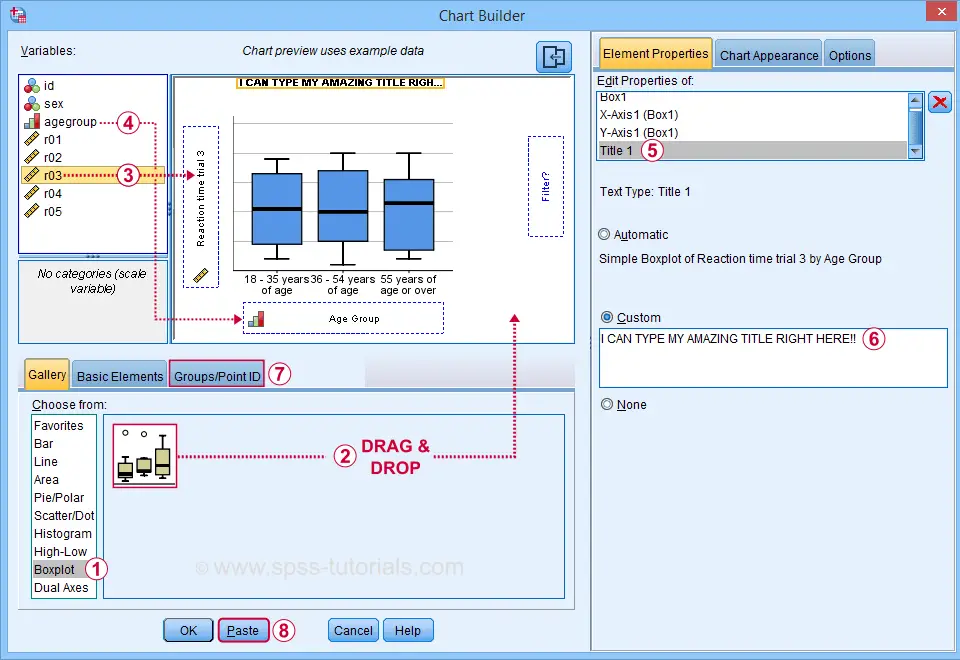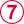Select “Point ID Label” in this tab and then drag & drop r03 into the ID box on the canvas. Doing so will show actual outlier values in the final boxplot.

Completing these steps results in the syntax below.

*Boxplot for comparing 3 age groups on 1 variable.

GGRAPH
/GRAPHDATASET NAME="graphdataset" VARIABLES=agegroup r03 MISSING=LISTWISE REPORTMISSING=NO
/GRAPHSPEC SOURCE=INLINE.
BEGIN GPL
SOURCE: s=userSource(id("graphdataset"))
DATA: agegroup=col(source(s), name("agegroup"), unit.category())
DATA: r03=col(source(s), name("r03"))
GUIDE: axis(dim(1), label("Age Group"))
GUIDE: axis(dim(2), label("Reaction time trial 3"))
GUIDE: text.title(label("I CAN TYPE MY AMAZING TITLE RIGHT HERE!"))
SCALE: cat(dim(1), include("1", "2", "3"))
SCALE: linear(dim(2), include(0))
ELEMENT: schema(position(bin.quantile.letter(agegroup*r03)), label(r03))
END GPL.

## Result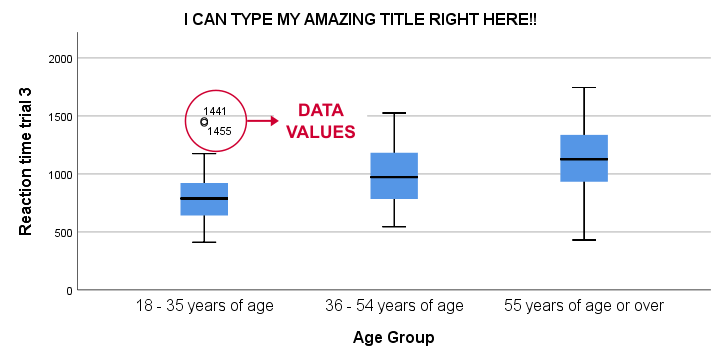This boxplot shows increasing medians and standard deviations with increasing ages. Note that our boxplot also shows outlier values. In this example, these are reaction times of 1,441 and 1,455 milliseconds but for the youngest age group only.

## Tip 1 - Remove Outliers for Single Group

If you'd like to remove outliers based on boxplot results, you'd normally set them as user missing values. For example, MISSING VALUES r03 (1441 THRU HI). sets values of 1441 and higher as missing for r03. In our example, however, this won't work: the aforementioned values are potential outliers only for the youngest age group. For the other age groups, they're within a normal range.

A solution is converting these values into different values for the youngest age group only. One option is combining DO IF with RECODE. The syntax below, however, shows a shorter option based on IF.

*Quick checktable.

means r03 by agegroup
/cells count min max mean stddev.

*Recode potential outliers into 999999998 but only for agegroup 1.

if(agegroup = 1 and r03 >= 1441) r03 = 999999998.

*Set recoded outliers as user missing values.

missing values r03 (999999998).

*Apply value label to recoded outliers.

add value labels r03 999999998 'Value removed because outlier'.

*Rerun checktable.

means r03 by agegroup
/cells count min max mean stddev.

## Tip 2 - Show Outlier Values in Boxplot

You can show data values for potential outliers and extreme values in boxplots. This only works if each boxplot involves a single dependent variable. Simply use this dependent variable as the ID variable too.
The only dialog that supports this is the Chart Builder. If you prefer the other dialogs, modifying the /ID subcommand in the syntax also does the trick.

*Show potential outlier values and extreme values -if any- in boxplot.

EXAMINE VARIABLES=r03 BY agegroup
/PLOT=BOXPLOT
/STATISTICS=NONE
/NOTOTAL
/ID=r03. /*Label outliers with actual data values.

## Tip 3 - Adding Titles to Boxplots

There's 3 options for showing titles in SPSS boxplots:

• create your boxplot via the Chart Builder as in example 3;
• use a chart template that has a fixed title and/or subtitle;

For this last option, open a Chart Editor window by double-clicking your chart. You can now add a title from the Options menu.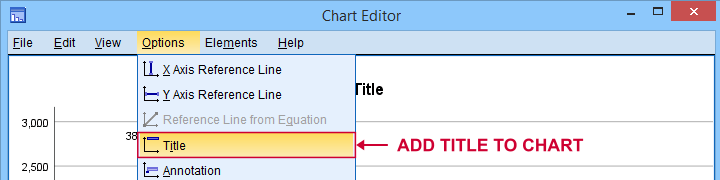## Final Notes

There's many more variations on boxplots, especially clustered boxplots. However, I think you'll get them done fairly easily after studying this tutorial.

If you've any questions or remarks, please throw me a comment below.

# Tell us what you think!

*Required field. Your comment will show up after approval from a moderator.

# THIS TUTORIAL HAS 9 COMMENTS:

•### By Ruben Geert van den Berg on May 4th, 2022

Hi Jon, those are interesting examples, especially the last one.

The R-chart seems to be some kind of bitmap (.bmp/.png?) object in the output window, I'd never seen this before.

But still...

I don't see why anybody would prefer a boxplot over a (split) histogram or a scatterplot.

A strong argument for the latter is that you could reconstruct the raw data almost perfectly from the chart visualizing them so they're very complete but still a single image.

I should add that I've become a bit of a CURVEFIT fan over the past year. Bummer that I don't have the time/funding to spend substantial time on it.

•### By Jon Peck on May 4th, 2022

Vase plots, which are boxplots with a mini histogram as the box sides don't seem to be very popular even though they provide a bit more information about the distribution.

The virtue of boxplots, though, is that they show summary information on the distribution - just like means and standard deviations do. There is value on the summaries, especially in a use like the residual boxplots case, where they provide visuals for heteroscedasticity and functional form problems where the extra detail of a vase plot would be a distraction. Dot plots as well as scatters provide more detail but don't scale very well and may sometimes obscure patterns.

There are many other variations on the boxplot. This link provides a bunch.
https://datavizcatalogue.com/blog/box-plot-variations/

I wrote a note on graphically validating regression assumptions. It is installed with the regression residual plots extension but can also be found here.

•### By Mrinal chadha on May 20th, 2022

Ultimate blog, very well articulated, and an easy step-by-step guide on SPSS. Thank you for sharing

•### By Jon K Peck on May 9th, 2023

More boxplot-like options:

- The Graphs > Regression Variable Plots (STATS REGRESS PLOT) extension command, which is intended to help screen potential regressors for a regression model, can produce sets of boxplots for the categorical variables included. This would be a collection of small plots.

- Bagplots, Graph > Bagplots (STATS BAGPLOTS), produces a matrix of two-dimensional bagplots or just a single bagplot.

- Boxplots have been generalized to show more information about a distribution. Graphs > Graphboard Template Chooser, can produce a violin plot that shapes the boxplot box according to the distribution, This requires a template that is shipped with Statistics 29, but for earlier versions, it can be downloaded from here